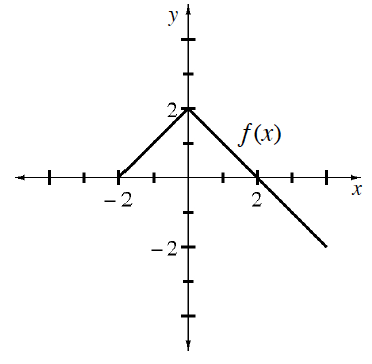### Home > APCALC > Chapter 8 > Lesson 8.1.4 > Problem8-46

8-46.

Given the graph of $y = f (x)$ at right, determine the values of $k$ that will make each integral expression equivalent to $\int _ { 0 } ^ { 2 } f ( x ) d x$.

1. $k \int _ { - 2 } ^ { 2 } f ( x ) d x$

1. $k \int _ { - 2 } ^ { 4 } f ( x ) d x$

1. $k \int _ { 2 } ^ { 4 } f ( x ) d x$

1. $k \int _ { 4 } ^ { 2 } f ( x ) d x$$\int_{0}^{2}f(x)dx=2$

These answers are in no particular order.
Match the answer to the problem:

$1 \ \ \ \ \ \ -1 \ \ \ \ \ \ \frac{1}{2} \ \ \ \ \ \ 1$